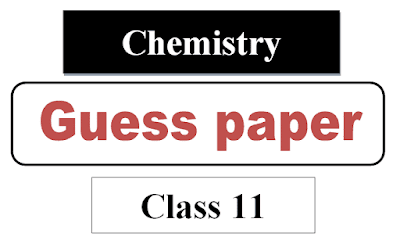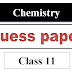This guess paper is for class 11 chemistry for exams 2022 according to 1st year chemistry syllabus 2022. The guess paper includes 1st year chemistry chapter wise important short questions and important long questions 2022.

# 1st year chemistry Guess paper 2022 Punjab boards

The Punjab boards like Lahore board, Multan board, Sahiwala board, Bhawalpur board, Sargodha board, DG khan board, faisalabad board, rawalpindi boards etc students can download this guess paper in pdf. The guess paper is very short document in pdf which consists of all important long questions and short questions for 1st year chemistry.

The list of important questions have been taken from chemitry class 11 book and the students can find this very short and good guess paper presented by zahid notes. The link to download pdf file is given below the image.

You may also like the following:

Here is the guess paper for class 11, 1st year chemistry for all punjab boards download in pdf below:Now the post goes further with the following content:

### 1st year chemistry important long questions 2022

1. What is Dalton’s law of partial pressure? Also discuss its application.

2. One mole of methane is maintained at 300 K . Its volume is 250 cm3 . Calculate the pressure exerted by the gas when it considered as an ideal gas?

3. Calculate the mass of 1 dm3 of NH3 gas at 30 oC and 100 mm/Hg pressure, considering the NH3 is behaving ideally?

4. Give eight postulates of kinetic molecular theory.

5. What is H-bonding? Discuss H-bonding in biological compounds.

6. How does the hydrogen bonding explain the formation of ice lesser density than liquid water.

7. Define liquid crystals. Discuss important uses of liquid crystal.

8. Write down the properties of ionic solids.

9. Explain Rutherford’s model of atom. Give its defects.

10. Define Quantum numbers. Discuss briefly Azimuthal Quantum number.

11. Give the different postulates of Bohr’s atomic model.

12. Write down the main postulates of valence shell electron pair repulsion theory and discuss the structure of NH3 with reference of this theory.

13. Briefly explain shapes of NH3 and H2O molecule according to hybridizing theory.

14. Why bond formation is not possible between two He atoms? Prove with molecular orbital theory.

`15. Describe bomb Calorimeter

16. Define and explain Hess’s law of constant heat summation with examples.

17. Define the following terms: Molality, Mole fraction and part per million.

18. What is Raoul’s law? Give its three statements.

19. Describe one method to determine the boiling point of elevation of solutes.

20. State rules for assigning oxidation number of elements with examples.

21. Write construction and working of voltaic cell.

22. Describe the electrolysis of molten sodium chloride and a concentrated solution of sodium chloride.

23. Give four industrial importance of electrolysis process in detail.

24. What is electrochemical series? Give its four applications?

25. How light and surface are affect the rate of reaction.

26. Write four characteristics of a catalyst.

27. How does Arrhenius equation help us to calculate the energy of activation of reaction?

28. Define Half-Life period and order of reaction. Describe half-life method to determine the order or reaction.

You may like the following relevant posts:

1. 1st year guess papers

2. 1st year notes pdf

3. 2nd year paper schemes pdf

4. 1st year paper pattern

5. 1st year date sheet punjab board

6. 1st year Roll number slip

1st year chemistry guess paper 2022 pdf downloadReviewed by Saif Ullah ZahidonJune 22, 2022Rating: 5

1.2.